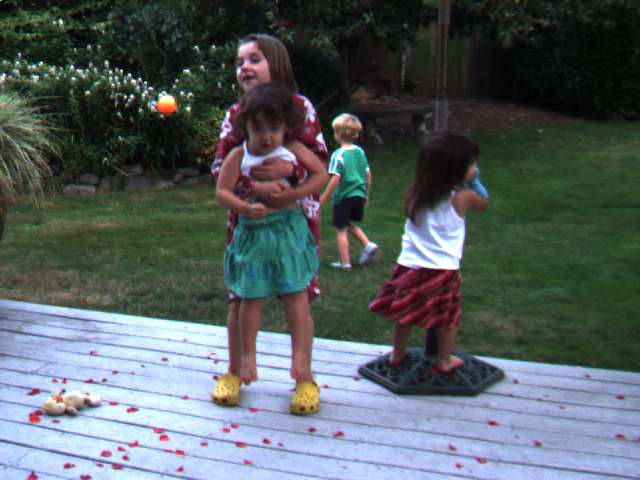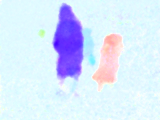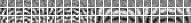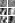## Optical flow with CNNs

Update August 2016:
if you do not have a GPU, replace the pretrained network (/results/net.mat) by this net.mat file. If you do not have the Matlab Parallel Computing toolbox installed, also put this dummy gather.m function in /misc/.

This package is a Matlab implementation of the following paper:
Learning to Extract Motion from Videos in Convolutional Neural Networks,
Damien Teney, Martial Hebert, arXiv Preprint [cs.CV], 2016.

It implements of a shallow, fully convolutional neural network (CNN) that takes consecutive frames of a video as input (typically 3), and extracts high-dimensional motion features, then typically projected as optical flow. The weights/filters of the network are learned by supervised training with standard backpropagation, using ground truth optical flow as labels.The network includes particular operations that allow training with small amounts of data (more details in the paper).

• Local whitening
We apply a center surround filter and a normalization by the local image variance. This is a local (since this network is fully convolutional) equivalent to the common (global) mean/variance preprocessing.
• Geometric invariance to rotation (covariance to be technically precise)
We define a number of discrete orientations (typically 12) and enforce, at every layer, groups of channels to represent features at each of these orientations. This is achieved by tying weights so that the same transformations are applied to each orientation. The explicit description of these "ties" is cumbersome, but the intuitive description is that:
(1) filters for different orientations are rotated versions of each other
(2) weights are forced to be the same when acting on channels (from the previous layer) representing the same *relative* orientation. In practice, only a small subset of independent weights need to be learned. This can also be interpreted as a (hard) regularizer on the weights.

 Weights/filters of the convolutional layersStructure is enforced by hard constraintsthat provide invariance to rotations. Corresponding independent weightsOnly a subset of weightshave to be actually learned.## How to use

The package contains:
- General functions to evaluate/train CNNs (inspired by MatConvNet).
- Compiled functions from MatConvNet implementing basic operations (convolutions, pooling, ...)
- Instantiation of our CNN-Flow architecture.
- Pregenerated results of these scripts (trained Matlab+Caffe networks).
- Example training/test data from the Middlebury optical flow dataset.

First, add all subdirectories to your Matlab path, then run one of the demo scripts:

demoCnnFlow_test.m will load the provided pretrained network and run the training/test sequences of the Middlebury dataset. It will display the ground truth flow (if available) and the estimated flow for comparison. It will also display the weights/filters of all convolutional layers (both the "reduced" set and the "complete" set of weights with the enforced rotation invariance).
demoCnnFlow_train.m contains 3 ways of training a network from the Middlebury dataset; see comments for details. Note that this will overwrite the provided pretrained network.
All the Matlab code can run on CPU or GPU (see parameter 'useGpu'). All functions are self-documented with extensive comments. The global wrapper flow() takes parameter/value pairs as arguments, then will call runCnn() and evalCnn() for training and/or testing. See flow_getParams() for the list of parameters. Some are defined below.

The dataset is defined as 3 splits (training/validation/test). 'runTrVaTe' defines which splits to process, and 'saveVisualizations' can enable saving and displaying the results of each split (the optical flow maps estimated by the network). For example:

flow('runTrVaTe', [1 10 0], 'saveVisualizations', [0 1 0], ...)
will run SGD training and evaluate the validation split every 10 epochs, while saving visualiations only of the validation set
flow('runTrVaTe', [0 0 1], 'saveVisualizations', [0 0 1], ...)
will only evaluate the network on the test split and save visualizations.

'continue' sets wether to initialize a new network or load a pretrained one (to continue training or to test it).

'randomInit' sets wether weights are initialized from random values, as hardcoded structured weights (as described in the paper, e.g. 3D Gaussian derivatives for the motion filters).

The parameter 'displayWeights'=true will display the weights of all convolutional layers (the full set of and the reduced set of independent weights that are actually learned).

'leftRightSplit' keeps the left/right halves of the images for training/ validation. We use this when there is not enough data to make distinct splits.

## Caffe model

We provide a function to export trained networks from our Matlab code to Caffe models. There are differences (simplifications) from the Matlab model imposed by making them useable without modifications to Caffe: - No recurrent/warping connection: reduced accuracy of motion estimates. - No special handling (e.g. mirror padding) of the borders for convolutions: motion estimates near the image borders are not correct. - No implementation of the weight tying for geometric invariances: Caffe models are intended for testing. Training/fine-tuning *might* be possible with a lot of data. Let us know if you try this ! - The multiscale processing only allows for integer factors; the dimensions of the input image should be divisible by the factors used.

We provide a script (demoCnnFlow_trainCaffeModel) that trains a simple model for a given input size, then save it as a Caffe model. This requires a local installation of Caffe with the MatCaffe interface.

We provide a ready-to-use Caffe model (the result of that script) as:
result/model1/cnnFlow.prototxt
result/model1/cnnFlow.caffemodel

As input, it takes 3 stacked grayscale frames. We provide a script (packThreeFrames.m) to pack 3 images into 1 RGB file that may be useful. It outputs a flow map at 1/4 the dimensions of the input.

Given that this network is fully convolutional, input dimensions can be adjusted (in the prototxt) without retraining. The only constraint is that the input dimensions must be divisble by the factors of the multiscale application. The script (see above) can be modified to retrain a network for another set of scaling factors. The input size in the prototxt can also be changed to process many sequences (triplets of images) at a time.

### Speed

The evaluation of the provided Caffe network, input size 256 x 256px, using 4 scales, runs in 0.025s (40 Hz) on a very modest laptop GPU (GeForce 930M).

## Re-compiling

We rely on MatConvNet for the core functions (convolutions, pooling, etc.). We included both the source files and precompiled binaries (MEX) for Linux/Windows, 64 bits, CUDA v7.5. For other configurations, you can recompile them as follows:
- In Matlab, go into the directory cnn/matconvnet
- run one of the following command:
vl_compilenn('EnableGpu', false)
vl_compilenn('EnableGpu', true)

Alternatively, or in case of problems, you can independently download and compile the latest version of MatConvNet and delete the cnn/matconvnet/ directory from this package.